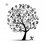# Is 0=1? Then find the mistake

Consider the integral, $\displaystyle I =\int \dfrac{1}{x}dx$

We know that $I= \log x$

But, $\displaystyle I =\int \dfrac{1}{x}(1)dx =(x)\dfrac{1}{x}-\int \bigg(-\dfrac{1}{x^2} \bigg) xdx$

$1+I=I \Rightarrow 1=0$

What is the mistake?

I do not know the mistakeNote by Sparsh Sarode
4 years, 6 months ago

This discussion board is a place to discuss our Daily Challenges and the math and science related to those challenges. Explanations are more than just a solution — they should explain the steps and thinking strategies that you used to obtain the solution. Comments should further the discussion of math and science.

When posting on Brilliant:

• Use the emojis to react to an explanation, whether you're congratulating a job well done , or just really confused .
• Ask specific questions about the challenge or the steps in somebody's explanation. Well-posed questions can add a lot to the discussion, but posting "I don't understand!" doesn't help anyone.
• Try to contribute something new to the discussion, whether it is an extension, generalization or other idea related to the challenge.

MarkdownAppears as
*italics* or _italics_ italics
**bold** or __bold__ bold
- bulleted- list
• bulleted
• list
1. numbered2. list
1. numbered
2. list
Note: you must add a full line of space before and after lists for them to show up correctly
paragraph 1paragraph 2

paragraph 1

paragraph 2

[example link](https://brilliant.org)example link
> This is a quote
This is a quote
    # I indented these lines
# 4 spaces, and now they show
# up as a code block.

print "hello world"
# I indented these lines
# 4 spaces, and now they show
# up as a code block.

print "hello world"
MathAppears as
Remember to wrap math in $$ ... $$ or $ ... $ to ensure proper formatting.
2 \times 3 $2 \times 3$
2^{34} $2^{34}$
a_{i-1} $a_{i-1}$
\frac{2}{3} $\frac{2}{3}$
\sqrt{2} $\sqrt{2}$
\sum_{i=1}^3 $\sum_{i=1}^3$
\sin \theta $\sin \theta$
\boxed{123} $\boxed{123}$

Sort by:

you actually got $\int \frac{1}{x} -\int \frac{1}{x}=1$. There is absolutely nothing wrong with that. Those are indefinite integrals.

- 4 years, 6 months ago

What if I add limits from 1 to 2..you still get 1=0

- 4 years, 6 months ago

- 4 years, 6 months ago

It is $\frac{x}{x} |_{a}^{b}$. That yeilds zero. 1 there has limits.

- 4 years, 6 months ago

I didn't quite get u, u meant $\dfrac{x}{x} =\dfrac{b-a}{b-a} \neq 1$

- 4 years, 6 months ago

I can't believe you just stated that...

- 4 years, 6 months ago

Correct me if I am wrong, isn't $\dfrac{2-1}{2-1}=\dfrac{1}{1}=1$ ?

- 4 years, 6 months ago

its $\frac{b}{b}-\frac{a}{a}$ and still $1|_a^b = 1_a-1_b=1-1=0$.

- 4 years, 6 months ago

Oops! I can't believe I made such a mistake... Anyways thx..

- 4 years, 6 months ago

- 4 years, 6 months ago

Can you post this as a problem so others can learn from this misconception?

Staff - 4 years, 6 months ago

Ok, surely

- 4 years, 6 months ago

Exactly... Then he should get correctly.

- 4 years, 6 months ago

How is LHS not equal to 0

- 4 years, 6 months ago

Integration constants need not be equal.

- 4 years, 6 months ago

K.. I agree on that

- 4 years, 6 months ago

And what happens without limits? $\displaystyle \int \frac{dx}{x} =\frac{x}{x}+\displaystyle \int \frac{dx}{x}$

- 4 years, 6 months ago

It's still correct.. Integration constants need not be same

- 4 years, 6 months ago

Oh, right, thank you

- 4 years, 6 months ago

Welcome.. :)

- 4 years, 6 months ago

You cannot cancel the integrals on both sides because each function has infinitely many anti-derivatives off by a constant. That's why, for good reason, you should always include "+C" in your answers.

- 3 years, 7 months ago